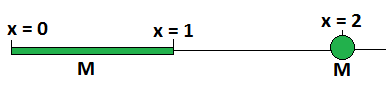# Gravitationally Equivalent Point ParticleThere are two objects with the same mass $M$, situated on the $x$-axis.

One is a thin rod with its mass uniformly distributed between $x = 0$ and $x = 1$.

The other is a point particle at $x = 2$.

If we wanted to replace the rod with a point particle of mass $M$ which would exert the same gravitational force on the point particle at $x = 2$, what should its $x$-coordinate be (to 3 decimal places)?

×